# Creating a Date table in Power BI

In this article, we will generate a date table in Power BI which will be a reference point for data models that can be used for time intelligence calculations.

Creating a date table is very easy with DAX functions. A date table is essential in creating data models. With a date table, we can create Tables, Charts & Slicers in reports.

Now let’s start building a Date table called Date master for 2021 -2022. This date table will include Date, Year, Month, Week, Day, Quarter, Weekday, Weeknumber, Day Name & Month Name by using DAX functions.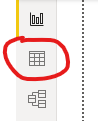Step-B: From the Table tools ribbon, click New table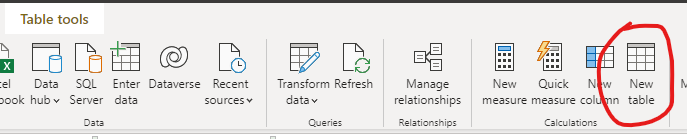Step-C: Once we click on the new table, we will get the option to write the function. Now let’s write a DAX measure like the below screenshot to generate the calendar of 2022 in the table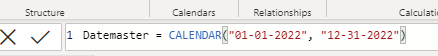Ex: Tablename = Calendar(“Startdate”, “Enddate”)

Step-D: The table will generate date in a single column, and Datemaster will appear in your data Fields.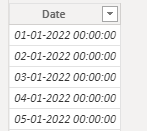Step-E: Click on the new column and write the Dax formula to generate Year, Month, WeekDay, Day-Name using DAX functions.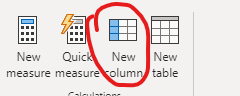Step-F: Let us create new columns with DAX measures by giving using formulas as shown below:

1) Year: Year = YEAR([Datemaster[Date])
DAX Formula: Year = YEAR(Tablename[Datecolumn])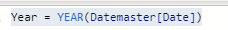2) Month : Month = MONTH([Datemaster[Date])
DAX Formula: Month = MONTH(Tablename[Datecolumn])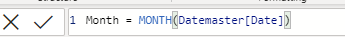3) Day: Day = DAY([Datemaster[Date])
DAX Formula: Day = DAY(Tablename[Datecolumn])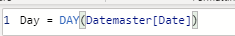4) WeekDay – To know which day (1-7) it belongs in a week.
Weekday = WEEKDAY([Datemaster[Date])
DAX Formula: weekday = WEEKDAY(Tablename[Datecolumn])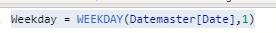5) Weeknum – To know the number of the week (1-52) it belongs in the year.
DAX Formula: Weeknum = WEEKNUM(Tablename[Datecolumn])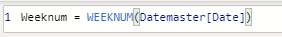6) Quarter – To know which quarter the week or month belongs in the year.
DAX Formula: Quarter = Quarter(Tablename[Datecolumn])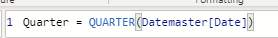7) Dayname – To know the day name of the week(Sunday – Saturday), We need to use the Format function to extract the date in word format. To get a full-day name, we need to use “DDDD” (Example: Sunday, Monday). If you need a short day name, then you can type “DDD” (Example: Sun, Mon)
DAX Formula: Dayname = FORMAT(Tablename[Datecolumn])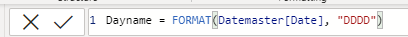8) MonthName – To know the month in word format (January – December), We need to use the Format function to extract the date in word format. To get the month name in full, we need to use “MMMM” (Example: January). If you need a short month name, use “MMM” which will give January as Jan/ April as Apr, etc.
DAX Formula: MonthName = FORMAT(Datemaster[Date], "MMMM")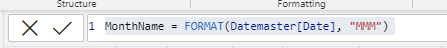Step-G: After creating a new table with all the above DAX formula, the table looks like this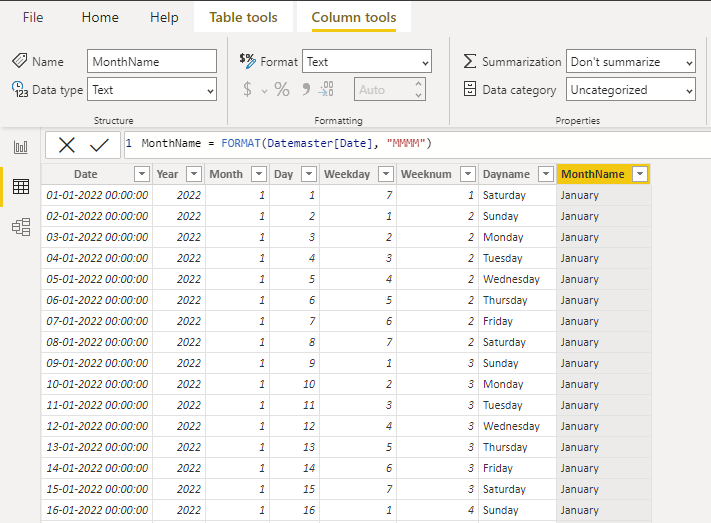Step-H: After creating the table, it’s essential that we need to map these tables with an existing table by linking the Date column. For example, the CR_Application table is used as a reference to link the Datemaster table, as shown below.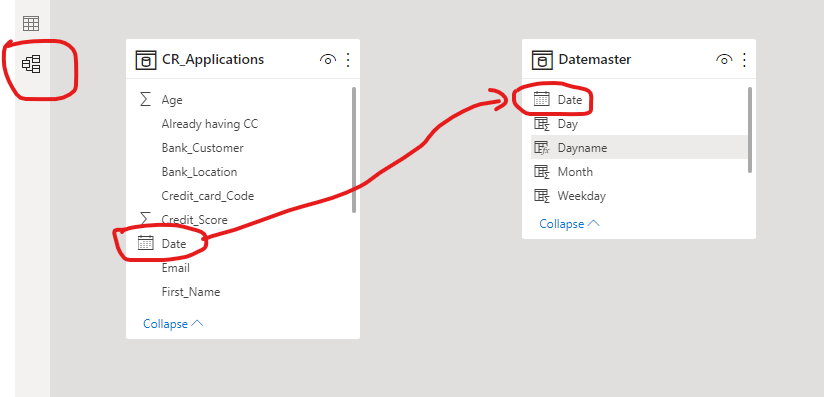Step-I: To link these two tables, we will drag and drop Date column from either of the tables. As can be seen in the image below, the dotted line connecting both column indicates that these two tables are now connected.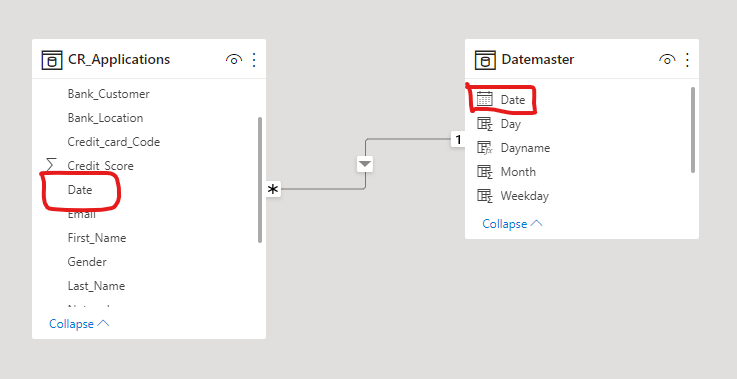Step-J: In the data Fields pane, we can see the newly created table (Datemaster) along with the existing table.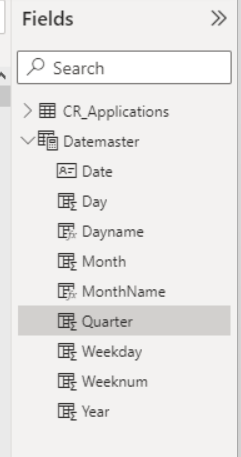Conclusion:

Creating a date table is easy, but it’s essential to understand that the DAX formula plays a vital role in showing the date in many different varieties. With the above DAX functions, we can create a date table that helps generate a Dashboard or reports. This is the standard procedure to create a date table, which can save you time in the query editor.Miss. Priya & Arun DN
Business analyst, Blogger and Tableau Trainer.